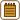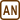## Bar Expansion During Its Cooling

### Task number: 2220

We heat up a steel bar with cross section diameter of 2 cm2 from temperature 0 °C to 50 °C. Then we cool it down back to its original temperature.

Calculate the smallest force acting on the axis of the bar which we would need to use to prevent the shortening of the bar. Young’s module is for simplicity considered a constant.

Note: Coefficients of thermal expansion of steel and iron are approximately the same.

• #### Hint

To prevent the shortening of the bar, we need to act on it with the same force as if we wanted to prolong it at 0 °C to the length it will have at 50 °C.

• #### Analysis

The prolonging of the heated bar is directly dependent on the temperature difference. The bar will shorten back to its original length during the cooling. If we want to prevent it, we have to act on it with the same force as if we wanted to prolong it from its original length to the length it will have at 50 °C. This prolonging follows Hooke’s law for deformation by stretching.

• #### List of Known Information

 S = 2 cm2 = 2·10−4 m2 bar’s cross section diameter t0 = 0 °C bar’s original temperature t1 = 50 °C bar’s temperature after heating up F = ? force that we have to act with

From tables:

 α = 12·10−6 °C−1 coefficient of thermal expansion of steel E = 21·1010 Pa Young’s module of steel
• #### Solution

The bar’s length increases when being heated up. This increasement Δl is directly dependent on the temperature difference Δt = t1t0 according to equation

$\mathrm{\Delta} l=l_0 \alpha\mathrm{\Delta}t =l_0\alpha(t_1-t_0),$

where l0 is the original length of the bar and α is the coefficient of thermal expansion.

The bar’s length should decrease back to the original length l0 after cooling down to the original temperature. If we want to prevent this, we have to strech the bar with a force equal to the force we would need to stretch it with if we wanted to lengthen it from length l0 to l0l.

Force F required to increase the relative length of the bar by ε is directly dependent on this elongation according to Hooke’s law:

$\sigma=E\varepsilon,$

where the constant of proportion is Young’s module of elasticity E and σ is normal tension caused by force F defined as

$\sigma=\frac{F}{S}$

and relative elongation ε is defined by relation

$\varepsilon=\frac{\mathrm{\Delta}l}{l_0}.$

We gain after substitution for σ and ε into Hooke’s law:

$\frac{F}{S}=E\,\varepsilon\qquad\Rightarrow\qquad F=E\,S\,\varepsilon=E\,S\,\frac{\mathrm{\Delta}l}{l_0}.$

Now we can substitute for Δl and get the final relation:

$F=E\,S\,\frac{\mathrm{\Delta}l}{l_0}=E\,S\,\frac{l_0\,\alpha\,\left(t_1-t_0\right)}{l_0}=E\,S\,\alpha\,\left(t_1-t_0\right).$

Note that the required force is independent of the bar’s length so it doesn’t matter that it wasn’t given.

Numerical substitution:

$F=E\,S\,\alpha\,\left(t_1-t_0\right)=21\cdot{10^{10}}\cdot2\cdot{10^{-4}}\cdot{12}\cdot{10^{-6}}\,\left(50-0\right)\,\mathrm{N}=$ $=25\,200\,\mathrm{N}=25.2\,\mathrm{kN}$
• #### Answer

We need to act on the bar with force of 25.2 kN to prevent it from shortening.